• MATLAB源码集锦-基于埃尔米特插值多项式代码
• 埃尔米特插值法 为了让插值函数能更好的和原来的函数重合，不但要求二者在节点上函数值相等，而且要求相切，对应导数也相等，甚至要求高阶导数也相等。...MATLAB中编程实现的埃尔米特插值法为Hermite。 用于...

埃尔米特插值法

为了让插值函数能更好的和原来的函数重合，不但要求二者在节点上函数值相等，而且要求相切，对应导数也相等，甚至要求高阶导数也相等。——这类插值被称为切触插值，或埃尔米特插值，满足这种要求的多项式被称为埃尔米特插值多项式。

表达式如下：（欢迎大家指点如何在blog上打出一些数学算符，这里是在ward上打的）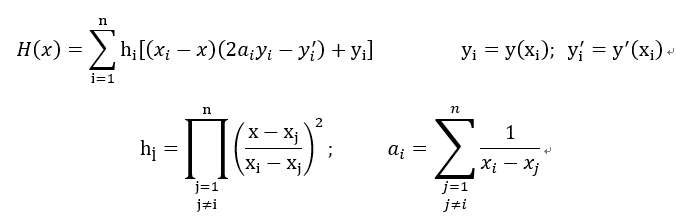正文：

• MATLAB中编程实现的埃尔米特插值法为Hermite。
• 用于求知数据点的埃尔米特插值多项式
• 调用格式：herm=Hermite（x,y,dy,x0）
• herm：求得的埃尔米特插值多项式或在x0处的插值
• x ： 数据x坐标向量
• y ： 数据y坐标向量
• dy ：已知数据点的导数向量
• x0 ：插值点的x坐标

在MATLAB上实现埃尔米特插值的程序如下：

function herm =Hermite(x,y,dy,x0)
format long  				%指定数据类型
syms t;        				%变量
get=0;         				%初值

if(length(x)==length(y))            %数据长度校验，可写可不写
if(length(y)==length(dy))
n_len=length(x);
else
return;
end
else
return;
end

for i=1:n_len                   		%开始描述表达式
h=1;
a=0;
for j=1:n_len
if(j~=i)
h=h*(t-x(j))^2/((x(i)-x(j))^2);
a=a+1/(x(i)-x(j));
end
end
get=get+h*((x(i)-t)*(2*a*y(i)-dy(i))+y(i));
if(i==n_len)
if(nargin==4)
get=subs(get,'t',x0);
else
get=vpa(get,6);
end
end
end
herm=get;
end

关于测试

网上普遍的例题：
求下表埃尔米特插值多项式，计算当x=1.44时y值

xyy’
0.510.5000
1.01.10.4
1.51.20.3
2.01.30.25
2.51.40.2

输入x，y，y’运行得到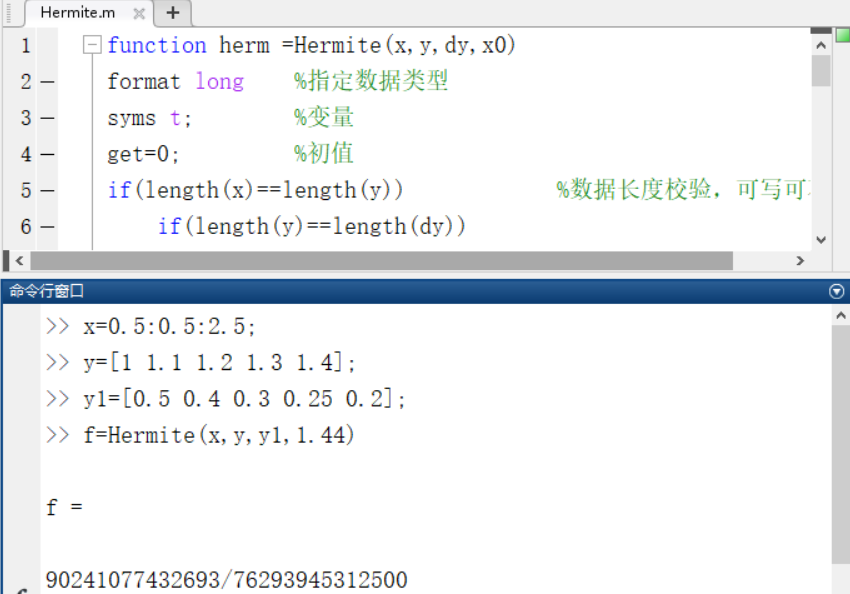展开全文MATLAB 埃尔米特插值
• %hermite.m%求埃尔米特多项式和误差估计的MATLAB主程序%输入的量:X是n+1个节点(x_i,y_i)(i = 1,2, ... , n+1)横坐标向量，Y是纵坐标向量，%以f'(x_i)=y'_i(i = 1,2,...,n+1)为元素的向量Y1;%x是以向量形式输入的m个...%hermite.m

%求埃尔米特多项式和误差估计的MATLAB主程序

%输入的量:X是n+1个节点(x_i,y_i)(i = 1,2, ... , n+1)横坐标向量，Y是纵坐标向量，

%以f'(x_i)=y'_i(i = 1,2,...,n+1)为元素的向量Y1;

%x是以向量形式输入的m个插值点，M在[a,b]上满足｜f~(2n+2)(x)｜≤M

%注：f~(2n+2)(x)表示f(x)的2n+2阶导数

%输出的量：向量y是向量x处的插值，误差限R,2n+1阶埃尔米特插值多项式H_k及其系数向量

%H_c,误差公式wcgs及其系数向量Cw.

function[y,R,Hc,Hk,wcgs,Cw] = hermite(X,Y,Y1,x,M)

n = length(X);

m = length(x);

for t = 1 : m

z = x(t);

H = 0;

q = 1;

c1 = 1;

for k = 1 : n

s = 0;

V = 1;

for i = 1 : n

if k ~= i

s = s + (1/(X(k)-X(i)));

V = conv(V,poly(X(i)))/(X(k)-X(i));

end

end

h = poly(X(k));

g = ([0 1]-2 * h * s);%注意不要写成1-2*h*s，因为是多项式减法，低阶多项式前面必须用零填补，书上的错误，浪费我一晚上时间

G = g * Y(k) + h * Y1(k);

H = H + conv(G,conv(V,V));%hermite插值多项式

b = poly(X(k));

b2 = conv(b,b);

q = conv(q,b2);

end

Hc = H;

Hk = poly2sym(H);

Q = poly2sym(q);

for i = 1 : 2*n

c1 = c1 * i;

end

wcgs = M * Q / c1;

Cw = M * q / c1;

y(t) = polyval(Hc,x(t));

R(t) = polyval(Cw,x(t));

end%hermite.m

%求埃尔米特多项式和误差估计的MATLAB主程序

%输入的量:X是n+1个节点(x_i,y_i)(i = 1,2, ... , n+1)横坐标向量，Y是纵坐标向量，

%以f'(x_i)=y'_i(i = 1,2,...,n+1)为元素的向量Y1;

%x是以向量形式输入的m个插值点，M在[a,b]上满足｜f~(2n+2)(x)｜≤M

%注：f~(2n+2)(x)表示f(x)的2n+2阶导数

%输出的量：向量y是向量x处的插值，误差限R,2n+1阶埃尔米特插值多项式H_k及其系数向量

%H_c,误差公式wcgs及其系数向量Cw.

function[y,R,Hc,Hk,wcgs,Cw] = hermite(X,Y,Y1,x,M)

n = length(X);

m = length(x);

for t = 1 : m

z = x(t);

H = 0;

q = 1;

c1 = 1;

for k = 1 : n

s = 0;

V = 1;

for i = 1 : n

if k ~= i

s = s + (1/(X(k)-X(i)));

V = conv(V,poly(X(i)))/(X(k)-X(i));

end

end

h = poly(X(k));

g = ([0 1]-2 * h * s);%注意不要写成1-2*h*s，因为是多项式减法，低阶多项式前面必须用零填补，书上的错误，浪费我一晚上时间

G = g * Y(k) + h * Y1(k);

H = H + conv(G,conv(V,V));%hermite插值多项式

b = poly(X(k));

b2 = conv(b,b);

q = conv(q,b2);

end

Hc = H;

Hk = poly2sym(H);

Q = poly2sym(q);

for i = 1 : 2*n

c1 = c1 * i;

end

wcgs = M * Q / c1;

Cw = M * q / c1;

y(t) = polyval(Hc,x(t));

R(t) = polyval(Cw,x(t));

end从这图片看要比拉格朗日和牛顿插值要好，不过当插值多了以后，也就是埃尔米特多项式的次数高了以后误差会变得很大的。不信我们来试试。

我们还是用sinx，不过这次我们用5个点。

X                       0                         pi/6                          pi/4                         pi/3                     pi/2

Y                       0                         0.5                          0.7071                    0.8660                    1

Y1                     1                        0.8660                     0.7071                       0.5                       0

>> X = [0 pi/6 pi/4 pi/3 pi/2];Y = [0 0.5 0.7071 0.8660 1];Y1 = [1 0.8660 0.7071 0.5 0];

>> M = 1;

>> x = linspace(0, pi, 50);

>> [y,R,Hc,Hk,wcgs,Cw] = hermite(X,Y,Y1,x,M)

>> y1 = sin(x);

>> errorbar(x,y,R,'.g')

>> hold on

>> plot(X, Y, 'or', x, y, '.k', x, y1, '-b');

>> legend('误差','样本点','埃尔米特插值估算','sin(x)');可以看到拟合的多项式从x=2.5开始远远偏离sinx，并且此时误差公式也失效了.这就是我们后面需要讲到的高次插值的振荡问题。

展开全文• ①数据插值可以根据有限个点的取值状况，合理估算出附近其他点的取值，从而节约大量的实验和测试资源，节省大量的人力、物力和财力。 ②数据插值能够根据已知数据推算未知数据，这使得人们解决问题的能力得到了拓展...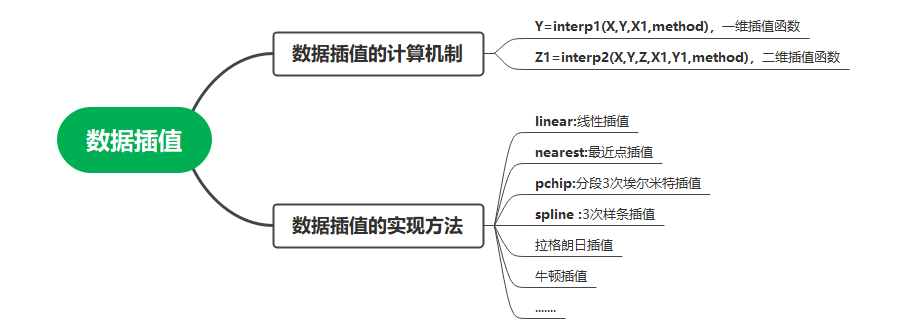①数据插值可以根据有限个点的取值状况，合理估算出附近其他点的取值，从而节约大量的实验和测试资源，节省大量的人力、物力和财力。
②数据插值能够根据已知数据推算未知数据，这使得人们解决问题的能力得到了拓展和延伸。

1、引例-零件加工问题

例1、在飞机制造中，机翼的加工是一项关键技术。由于机翼尺寸很大，通常在图纸中只能标出一些关键点的数据。下表给出了某型飞机机翼的下缘轮廓线数据，求x每改变0.1时y的值。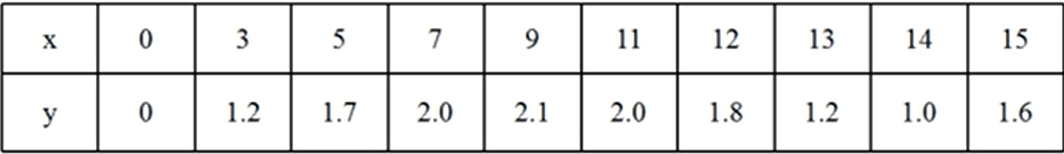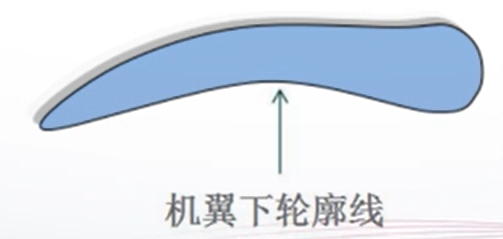它前段采样点稀疏，后段采样点密集，说明这段曲线前面可能比较规律、平滑，后段比较复杂

x = [0 3 5 7 9 11 12 13 14 15];
y = [0 1.2 1.7 2 2.1 2 1.8 1.2 1 1.6];
plot(x,y)    %为插值前的曲线
hold on;
x1 = 0:0.1:15;    %x每改变0.1要插一个值
y1 = interp1(x,y,x1,'spline');    %插值函数interp1:计算出这些插值点在y方向上的值存入y1中,y1很长
plot(x1,y1)   %完成插值后的曲线，曲线变得光滑很多
legend('y原曲线','y1插值后的曲线')
title('零件加工问题','color','r','fontsize',18)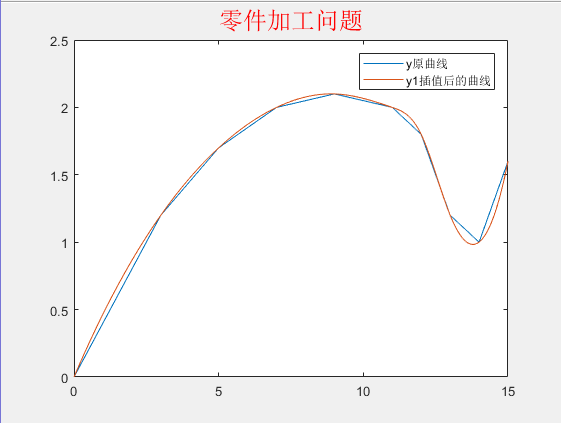2、数据插值的计算机制
从数学上来说，数据插值是一种函数逼近的方法。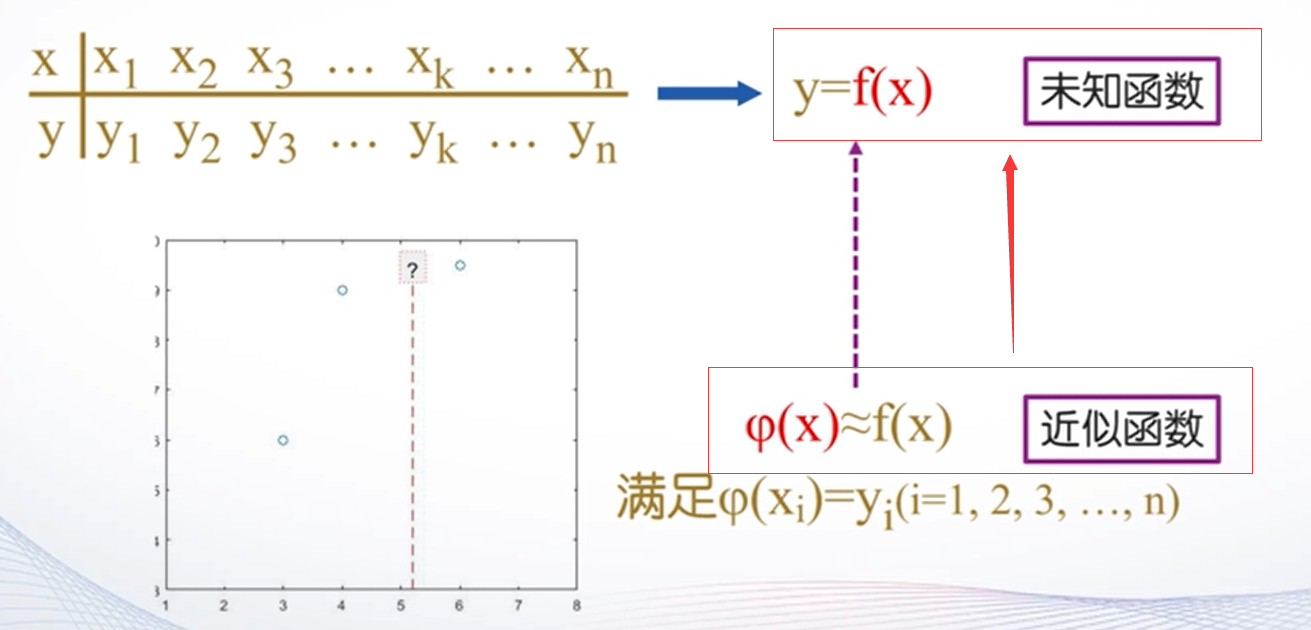（1）interp1( ):一维插值函数。调用格式:
Y=interp1(X,Y,X1,method)
根据X、Y的值,计算函数在×1处的值。其中，X、Y是两个等长的已知向量,分别表示采样点和采样值。X是一个向量或标量,表示要插值的点。

3、数据插值的实现方法
（1）一维插值method用于指定插值方法，常用的取值有以下四种:
①linear:线性插值，默认方法。
将与插值点靠近的两个数据点用直线连接，然后在直线上选取对应插值点的数据。
②nearest:最近点插值。
选择最近样本点的值作为插值数据。如果是中间点，则取后一个数据点的值。
③pchip:分段3次埃尔米特插值。
采用分段三次多项式，除满足插值条件，还需满足在若干节点处相邻段插值函数的一阶导数相等，使得曲线光滑的同时，还具有保形性。
④ spline :3次样条插值。
每个分段内构造一个三次多项式，使其插值函数除满足插值条件外，还要求在各节点处具有连续的一阶和二阶导数。（进—步提高了曲线的光滑性）

例2：以例1为例，进行四种数据插值的实现

x = [0 3 5 7 9 11 12 13 14 15];
y = [0 1.2 1.7 2 2.1 2 1.8 1.2 1 1.6];
x1 = 0:0.1:15;    %x每改变0.1要插一个值
% plot(x,y)
% hold on
subplot(2,2,1)
y1 = interp1(x,y,x1,'linear');    %插值函数interp1:计算出这些插值点在y方向上的值存入y1中,y1很长
plot(x,y,x1,y1)   %完成插值后的曲线
xlabel('interp1(x,y,x1,''linear'')','color','b')
legend('y','y1','location','northwest')
title('method = ''linear''','color','r','fontsize',14)

subplot(2,2,2)
y1 = interp1(x,y,x1,'nearest');    %插值函数interp1:计算出这些插值点在y方向上的值存入y1中,y1很长
plot(x,y,x1,y1)   %完成插值后的曲线
xlabel('interp1(x,y,x1,''nearest'')','color','b')
legend('y','y1','location','northwest')
title('method = ''nearest''','color','r','fontsize',14)

subplot(2,2,3)
y1 = interp1(x,y,x1,'pchip');    %插值函数interp1:计算出这些插值点在y方向上的值存入y1中,y1很长
plot(x,y,x1,y1)   %完成插值后的曲线
xlabel('interp1(x,y,x1,''pchip'')','color','b')
legend('y','y1','location','northwest')
title('method = ''pchip''','color','r','fontsize',14)

subplot(2,2,4)
y1 = interp1(x,y,x1,'spline');    %插值函数interp1:计算出这些插值点在y方向上的值存入y1中,y1很长
plot(x,y,x1,y1)   %完成插值后的曲线
xlabel('interp1(x,y,x1,''spline'')','color','b')
legend('y','y1','location','northwest')
title('method = ''spline''','color','r','fontsize',14)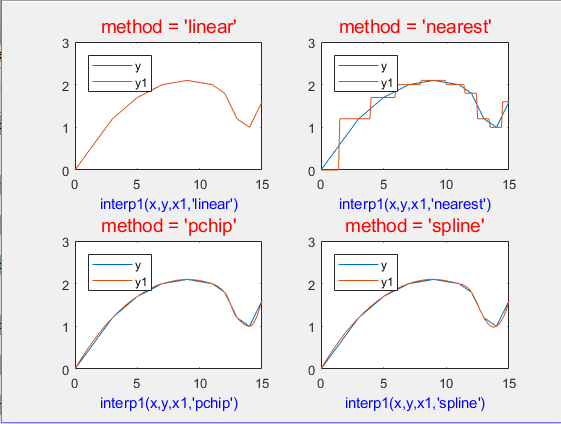四种方法的比较:
（1）线性插值和最近点插值方法比较简单。其中线性插值方法的计算量与样本点n无关。n越大，误差越小。
（2）3次埃尔米特插值和3次样条插值都能保证曲线的光滑性。相比较而言,3次埃尔米特插值具有保形性;而3次样条插值要求其二阶导数也连续,所以插值函数的性态更好。

（2）interp2():二维插值函数。调用格式:
Z1=interp2(X,Y,Z,X1,Y1,method)

其中，X、Y是两个向量，表示两个参数的采样点,Z是采样点对应的函数值。X1、YI是两个标量或向量，表示要插值的点。method与一维插值方法相同，但不支持pchip方法。

为什么这两种插值方法都用3次多项式而不用更高次的?
答：多项式次数并非越高越好。次数越高，越容易产生震荡而偏离原函数,这种现象称为龙格（Runge)现象。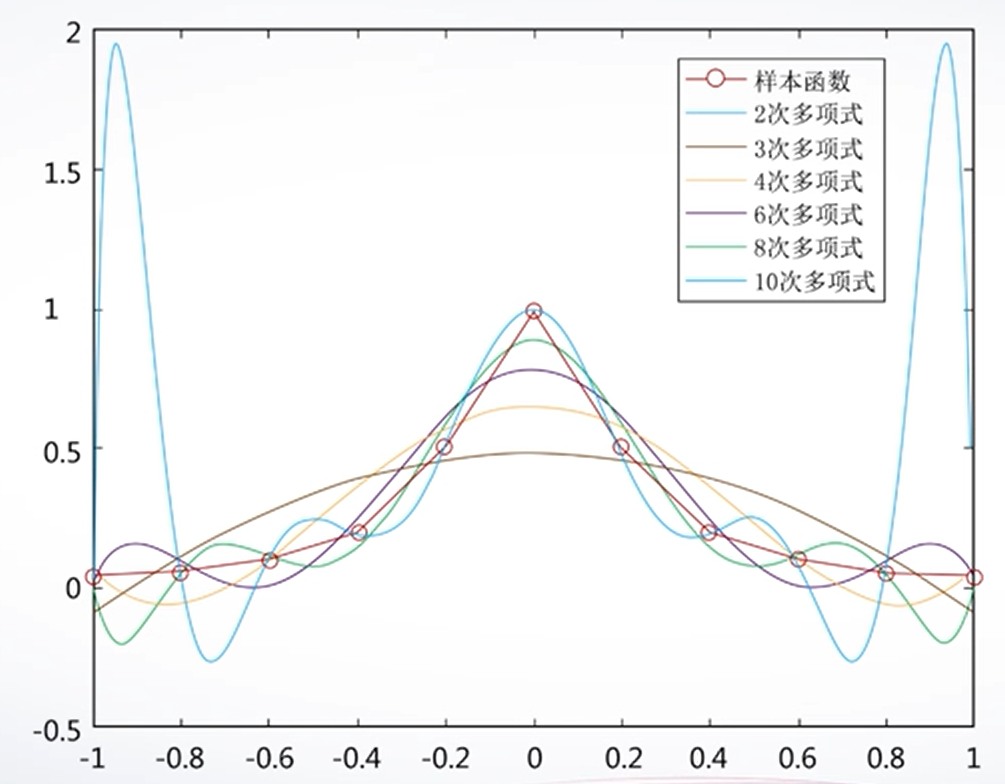展开全文matlab 数据插值
• Hermite插值满足在节点上等于给定函数值，而且在节点上的导数值也等于给定的导数值。对于高阶导数的情况，Hermite插值多项式比较复杂，在实际情况中，常常遇到的是函...matlab中的实现：Hermite.mfunction f = Herm...

Hermite插值满足在节点上等于给定函数值，而且在节点上的导数值也等于给定的导数值。对于高阶导数的情况，Hermite插值多项式比较复杂，在实际情况中，常常遇到的是函数值与一阶导数给定的情况。在此情况下，n个节点x1,x2,…,xn的Hermite插值多项式的表达式如下：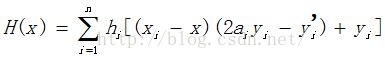其中：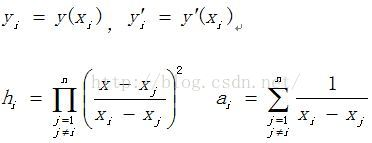这样就很容易写出代码了，关键就是记住公式。

matlab中的实现：

Hermite.m

function f = Hermite(x,y,y_1,x0)

%求已知数据点的向后差分牛顿插值多项式

%已知数据点的x 坐标向量:x

%已知数据点的y 坐标向量:y

%已知数据点的导数向量:y_1

%求得的Hermite插值多项式或x0处的插值:f

syms t;

f = 0.0;

if(length(x) == length(y))

if(length(y) == length(y_1))

n = length(x);

else

disp('y和y的导数的维数不相等！');

return;

end

else

disp('x和y的维数不相等！');

return;

end

for i=1:n

h = 1.0;

a = 0.0;

%%计算hi和ai

for j=1:n

if( j ~= i)

h = h*(t-x(j))^2/((x(i)-x(j))^2);

a = a + 1/(x(i)-x(j));

end

end

f = f + h*((x(i)-t)*(2*a*y(i)-y_1(i))+y(i));

if(i==n)

if(nargin == 4)

f = subs(f,'t',x0);

else

f = vpa(f,6);

end

end

end

HermiteInsert.m

x=1:0.2:1.8;

y=[1 1.0954 1.1832 1.2649 1.3416];

y_1=[0.5 0.4564 0.4226 0.3953 0.3727];

f=Hermite(x,y,y_1)

f=Hermite(x,y,y_1,1.44)

x1=0:2*pi;

y1=sin(x1);

y1_1=cos(x1);

xx=0:0.5:2*pi;

yy=Hermite(x1,y1,y1_1,xx);

plot(x1,y1,'o',xx,yy,'r')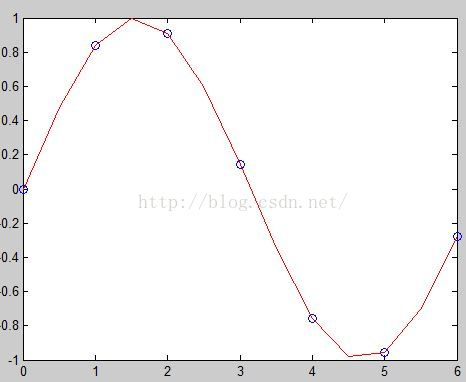本文同步分享在 博客“风翼冰舟”(CSDN)。

如有侵权，请联系 support@oschina.cn 删除。

本文参与“OSC源创计划”，欢迎正在阅读的你也加入，一起分享。

展开全文• 常常需要根据已知的函数点进行数据、模型的处理和 分析，而有时候现有的数据是极少的，不足以支撑分析的进行，这时就需要使用一些数学的方法，“模拟产生”一些新的但又比较靠谱的值来满足需求，这就是插值的作用。...插值算法 数学建模 三次样条插值 埃尔米特插值
• 《hermite插值以及两种MATLAB程序》由会员分享，可在线阅读，更多相关《hermite插值以及两种MATLAB程序(4页珍藏版)》请在人人文库网上搜索。1、给定矢量P0, P1, R0, R1,称满足下列条件的参数三次多项式曲线Pt,t0,1为...
• Hermite插值满足在节点上等于给定函数值，而且在节点上的导数值也等于给定的导数值。对于高阶导数的情况，Hermite插值多项式比较复杂，在实际情况中，常常遇到的是函...matlab中的实现：Hermite.mfunction f = Herm...
• 分段三次埃尔米特插值 % 分段三次埃尔米特插值 x = -pi:pi; %导入数据 y = sin(x); new_x = -pi:0.1:pi; p = pchip(x,y,new_x); figure(1);%同一脚本文件，想要画多个图，给每个图编号 plot(x,y,'o',new_x,p,'r-') ...matlab 机器学习
• 数据插值，拉格朗日，牛顿，埃尔米特插值，等距节点插值三次样条插值三次样条插值与拉格朗日的比较matlab
• 研究生数值分析课程_最全python插值程序(朗格朗日_埃尔米特_三次样条)_python。自己做的，南大的在读研究生。
• Matlab艾尔米特插值

千次阅读 2016-11-04 17:42:17
• 今天学习了插值法的matlab实现。 我们接触过五种基本的插值方法，有拉格朗日插值、牛顿插值、分段线性插值、分段三次Hermite插值和样条插值（三次）。 插值法在数学建模中的应用：数模比赛中，常常需要根据已知的...数学建模
• Hermite（埃尔米特）插值法

千次阅读 2021-01-26 10:23:25
Hermite（埃尔米特插值法 Hermite插值法是解决数学建模中预测类问题的最常用的方法，可以有效的解决“已知数据”数量不够的问题。 但是，直接使用Hermite插值得到的多项式次数较高，也存在着“龙格现象（Runge ...算法 matlab 数学建模
• 埃尔米特插值

2012-05-12 11:06:02
一直在一种曲线公式，过某些点，且在这些点的一阶导数、二阶导数有某些特性，网上找到了这个，基于承袭性，要比基函数要好，
• 实验要求： %alpha函数定义 function [res] = alpha(n,j) X = [0.1 0.2 0.3 0.4 0.5 0.6 0.7 0.8 0.9 1.0]; u = [-2*under(n,j) (2*X(j)*under(n,j)+1)];% ok!!... v = conv(base(n,j),base(n,j));...matlab
• 插值算法 一般定义 若P(x)为次数不超过n的代数多项式，即 P(x)=a0+a1x+...+anxn P(x)=a_0+a_1x+...+a_nx^n P(x)=a0​+a1​x+...+an​xn 若P(x)为分段多项式，即分段插值 若P(x)为三角多项式，即三角插值（一般用到...
• 求一个y=x^4的埃尔米特插值及误差的matlab编程 求一个y=x^4的埃尔米特插值及误差的matlab编程 求一个y=x^4的埃尔米特插值及误差的matlab编程matlab
• 目前有一组点，需要以插值的方式连成曲线，其中一部分点包含导数信息，其它点则没有，这样的算法应该以什么样的思路去编写呢？开发语言 开发语言 开发语言
• 两点三次埃尔米特插值法

千次阅读 2013-08-15 00:19:30
怎样用画笔画光滑曲线-两点三次埃尔米特插值法（转自http://blog.sina.com.cn/s/blog_a8192bdd010105rx.html） 求几点（3点以上）之间的光滑曲线是有很多办法的，如果需要曲线通过目标点，可以用经典的三次样条...
• MATLAB之埃米特插值

千次阅读 2020-04-15 11:07:51
此类问题被称为埃米特插值，相应的插值多项式成为埃米特插值多项式。 2、考虑函数值与导数值个数相等的情况 设在节点a≤x0<x1<...<xn≤b上,yi=f(xi),mi=f'(xi)(i=0、1...n) 要求插值多项式...matlab 数学建模 算法
• 1、拉格朗日插值新建如下函数：function y=lagrange(x0,y0,x)%拉格朗日插值函数%n 个节点数据以数组 x0, y0 输入(注意 Matlat 的数组下标从1开始),%m 个插值点以数组 x 输入,输出数组 y 为 m 个插值n=length(x0);...
• 1. 拉格朗日多项式插值了解概念插值多项式插值节点范德蒙特(Vandermonde)行列式截断误差、插值余项特点函数实现function y=lagrange(x0,y0,x)n=length(x0);m=length(x);for i=1:mz=x(i);s=0.0;for k=1:np=1.0;for j=...
• Matlab实现插值与拟合

千次阅读 2018-01-25 23:10:01
1、拉格朗日插值 新建如下函数： function y=lagrange(x0,y0,x) %拉格朗日插值函数 ...%m 个插值点以数组 x 输入,输出数组 y 为 m 个插值 n=length(x0);m=length(x); for i=1:m z=x(i); s
• 分段三次Hermite插值Matlab实现

万次阅读 2017-11-03 00:20:15
分段三次Hermite插值Matlab实现详解。matlab
• 1.埃尔米特(Hermite)插值1.1.Hermite插值多项式如果对插值函数，不仅要求它在节点处与函数同值，而且要求它与函数有相同的一阶、二阶甚至更高阶的导数值，这就是 Hermite 插值问题。本节主要讨论在节点处插值函数与......

matlab埃尔米特插值matlab 订阅